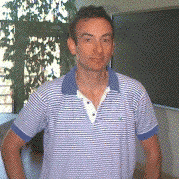# 2nd Annual Cantrell Lecture SeriesDr. Kenneth A. Ribet
University of California at Berkeley
Date and time:
Physics, Room 202

#### Professor Kenneth A. Ribet, University of California at Berkeley

Wednesday, November 8, 1995, 4:30 p.m.
Physics Building, Room 202

"Fermat's Last Theorem"

In the seventeenth century, Pierre de Fermat, a judge in Toulouse, wrote in the margin of a book that he had found a "marvelous proof" of a deceptively simple mathematical assertion. While there are many solutions in positive integers to the equation a2 + b2 = c2, Fermat claimed that there are no non-zero integers a, b and c satisfying a3 + b3 = c3 or a4 + b4 = c4. Moreover, Fermat said that no perfect nth power (with n bigger than 2) could be decomposed as a sum of two smaller nth powers. Unfortunately, Fermat wrote that the margin in which he was writing was too small to contain the proof.

For 350 years, researchers expended enormous effort trying to recover Fermat 's, or any other, "truly marvelous proof" of what came to be known as Fermat's "Last" Theorem. (It was the last assertion of Fermat to be proved.) In 1985, the German mathematician G. Frey pointed out a simple link between Fermat's equation and elliptic curves which suggested new lines of attack on Fermat's assertion. Two years ago, the Princeton mathematician Andrew Wiles announced that he had completed a proof of Fermat's Last Theorem which was based on Frey's construction.

In my lecture, I will explain the broad lines of the proof. In particular, I will describe how Fermat's Last Theorem became linked to the extraordinary Shimura-Taniyama conjecture, a statement about elliptic curves which became part of the mathematical landscape in the 1960s and '70s. After I proved that this conjecture implies Fermat's Last Theorem, Wiles set himself the task of proving the Shimura-Taniyama conjecture, despite the received wisdom that it could not be proved by currently available techniques. However, in an astounding breakthrough, the Shimura-Taniyama conjecture was recently proved (for a large class of elliptic curves) following ideas proposed by Andrew Wiles in 1993 (and then corrected and shortened in joint work of Richard Taylor and Wiles). As a consequence, Fermat's Last Theorem is now known to be true.

Thursday, November 9, 1995, 4:30 p.m.
Boyd Graduate Studies Research Center, Room 328

"Perfect powers in arithmetic progression"

The numbers 49, 169 and 289 represent three perfect squares in arithmetic progression. According to Fermat and Euler, there is no non-trivial four-term arithmetic progression consisting of perfect squares. Legendre proved that there is no three-term arithrnetic progression consisting of distinct positive cubes; Euler proved an analogous result for perfect fourth powers. What about higher powers? For perfect pth powers, the methods used to prove Fermat's Last Theorem show that three distinct pth powers cannot be in arithmetic progression if p is congruent to 1 mod 4. For p º 3 mod 4, we run up against open questions involving elliptic curves and modular curves.

Friday, November 10, 1995 4:00 p.m.
Boyd Graduate Studies Research Center, Room 328

"Component groups and degrees of modular parametrizations"

I shall report on a conjectural formula of Bertolini-Darmon and its proof in certain cases. Specifically, suppose that E is an elliptic curve over Q whose conductor is the product of two distinct primes. Assume further that E is the unique curve in its isogeny class. (Curves satisfying these conditions may be found in tables - the first example is the curve denoted 57E in the tables of Antwerp IV.) Then E is a quotient of two modular curves: the standard modular curve XO(pq) and the Shimura curve defined by the quaternion division algebra of discriminant pq. A formula compares the minimal degree of maps XO(pq) ® E and the minimal degree of maps X ® E.